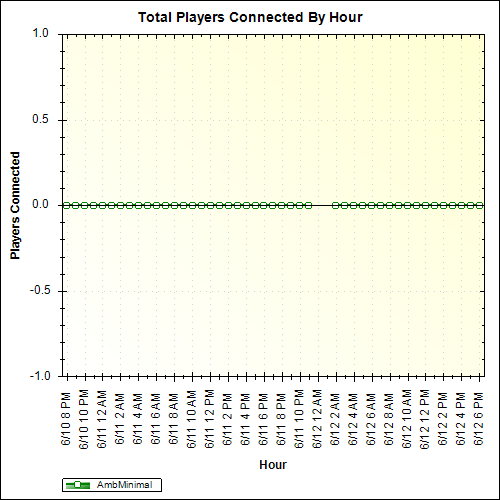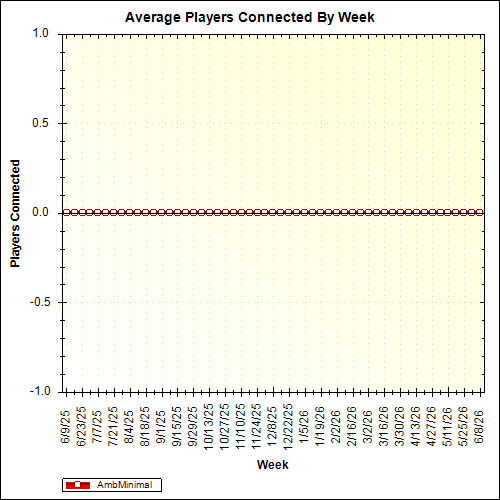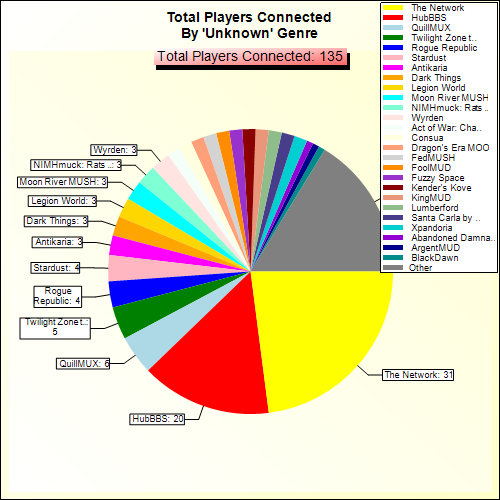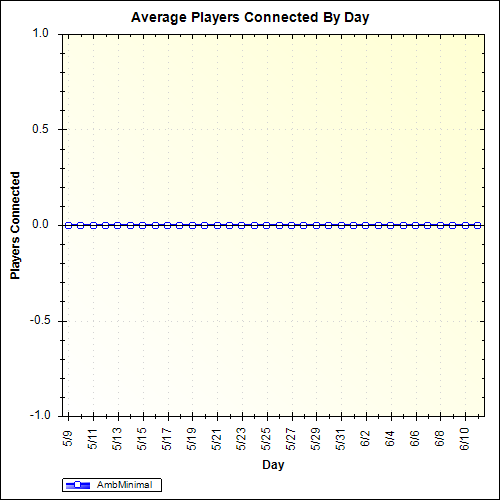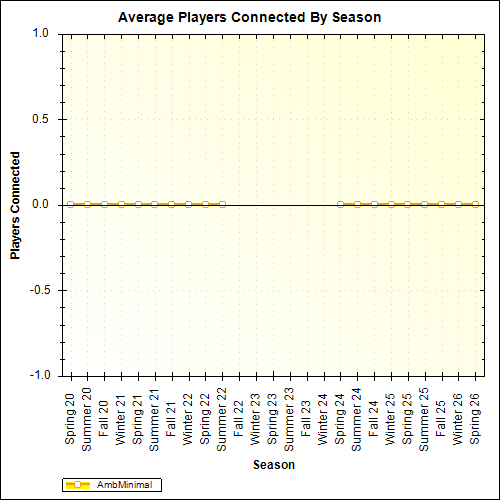AmbMinimal
Unknown-themed MUSH (Multi-User Shared Hallucination).
Ranked 715th of 834 worlds statistically.
Ranked 29th of 47 worlds in the Unknown genre statistically.

Db Size:
6797

Players Connected:
0 (an hour ago)

Maximum Connected:
0 (last 30 days)
Status:
UP

Version:
RhostMUSH Alpha 4.0.0RL(A)

Average Connected:
0 (last 30 days)

Minimum Connected:
0 (last 30 days)
Connection Screen
Welcome to RhostMUSH

/~\=/~\=/~\=/~\=/~\=/~\=/~\=/~\=/~\=/~\=/~\=/~\=/~\=/~\=/~\=/~\=/~\=/~\=/~\
((  To create new character     ----------=>   create <player> <password>  ))
)) To continue old character   ----------=>   connect <player> <password>((
((  To connect a guest          ----------=>   connect guest guest         ))
)) To see who is on            ----------=>   WHO                        ((
((  To quit your character      ----------=>   QUIT                        ))
)) To get help once connected  ----------=>   help (i.e. : help commands)((
((                                                                         ))
)) Note: use double quotes around name for names with spaces.            ((
((  Example: cr "player with spaces" password                              ))
\_/=\_/=\_/=\_/=\_/=\_/=\_/=\_/=\_/=\_/=\_/=\_/=\_/=\_/=\_/=\_/=\_/=\_/=\_/
~   ~   ~   ~   ~   ~   ~   ~   ~   ~   ~   ~   ~   ~   ~   ~   ~   ~   ~